This notebook contains an excerpt from the Python Data Science Handbook by Jake VanderPlas; the content is available on GitHub.

The text is released under the CC-BY-NC-ND license, and code is released under the MIT license. If you find this content useful, please consider supporting the work by buying the book!

No changes were made to the contents of this notebook from the original.

# Text and Annotation¶

Creating a good visualization involves guiding the reader so that the figure tells a story. In some cases, this story can be told in an entirely visual manner, without the need for added text, but in others, small textual cues and labels are necessary. Perhaps the most basic types of annotations you will use are axes labels and titles, but the options go beyond this. Let's take a look at some data and how we might visualize and annotate it to help convey interesting information. We'll start by setting up the notebook for plotting and importing the functions we will use:

In :
%matplotlib inline
import matplotlib.pyplot as plt
import matplotlib as mpl
plt.style.use('seaborn-whitegrid')
import numpy as np
import pandas as pd


## Example: Effect of Holidays on US Births¶

Let's return to some data we worked with earler, in "Example: Birthrate Data", where we generated a plot of average births over the course of the calendar year; as already mentioned, that this data can be downloaded at https://raw.githubusercontent.com/jakevdp/data-CDCbirths/master/births.csv.

We'll start with the same cleaning procedure we used there, and plot the results:

In :
births = pd.read_csv('data/births.csv')

quartiles = np.percentile(births['births'], [25, 50, 75])
mu, sig = quartiles, 0.74 * (quartiles - quartiles)
births = births.query('(births > @mu - 5 * @sig) & (births < @mu + 5 * @sig)')

births['day'] = births['day'].astype(int)

births.index = pd.to_datetime(10000 * births.year +
100 * births.month +
births.day, format='%Y%m%d')
births_by_date = births.pivot_table('births',
[births.index.month, births.index.day])
births_by_date.index = [pd.datetime(2012, month, day)
for (month, day) in births_by_date.index]

In :
fig, ax = plt.subplots(figsize=(12, 4))
births_by_date.plot(ax=ax);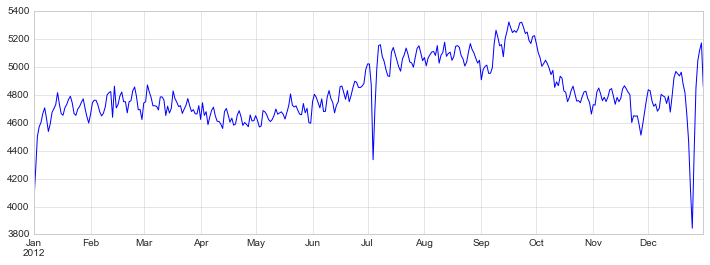When we're communicating data like this, it is often useful to annotate certain features of the plot to draw the reader's attention. This can be done manually with the plt.text/ax.text command, which will place text at a particular x/y value:

In :
fig, ax = plt.subplots(figsize=(12, 4))
births_by_date.plot(ax=ax)

# Add labels to the plot
style = dict(size=10, color='gray')

ax.text('2012-1-1', 3950, "New Year's Day", **style)
ax.text('2012-7-4', 4250, "Independence Day", ha='center', **style)
ax.text('2012-9-4', 4850, "Labor Day", ha='center', **style)
ax.text('2012-10-31', 4600, "Halloween", ha='right', **style)
ax.text('2012-11-25', 4450, "Thanksgiving", ha='center', **style)
ax.text('2012-12-25', 3850, "Christmas ", ha='right', **style)

# Label the axes
ax.set(title='USA births by day of year (1969-1988)',
ylabel='average daily births')

# Format the x axis with centered month labels
ax.xaxis.set_major_locator(mpl.dates.MonthLocator())
ax.xaxis.set_minor_locator(mpl.dates.MonthLocator(bymonthday=15))
ax.xaxis.set_major_formatter(plt.NullFormatter())
ax.xaxis.set_minor_formatter(mpl.dates.DateFormatter('%h'));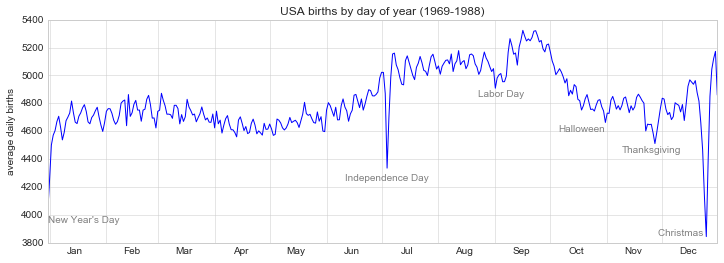The ax.text method takes an x position, a y position, a string, and then optional keywords specifying the color, size, style, alignment, and other properties of the text. Here we used ha='right' and ha='center', where ha is short for horizonal alignment. See the docstring of plt.text() and of mpl.text.Text() for more information on available options.

## Transforms and Text Position¶

In the previous example, we have anchored our text annotations to data locations. Sometimes it's preferable to anchor the text to a position on the axes or figure, independent of the data. In Matplotlib, this is done by modifying the transform.

Any graphics display framework needs some scheme for translating between coordinate systems. For example, a data point at $(x, y) = (1, 1)$ needs to somehow be represented at a certain location on the figure, which in turn needs to be represented in pixels on the screen. Mathematically, such coordinate transformations are relatively straightforward, and Matplotlib has a well-developed set of tools that it uses internally to perform them (these tools can be explored in the matplotlib.transforms submodule).

The average user rarely needs to worry about the details of these transforms, but it is helpful knowledge to have when considering the placement of text on a figure. There are three pre-defined transforms that can be useful in this situation:

• ax.transData: Transform associated with data coordinates
• ax.transAxes: Transform associated with the axes (in units of axes dimensions)
• fig.transFigure: Transform associated with the figure (in units of figure dimensions)

Here let's look at an example of drawing text at various locations using these transforms:

In :
fig, ax = plt.subplots(facecolor='lightgray')
ax.axis([0, 10, 0, 10])

# transform=ax.transData is the default, but we'll specify it anyway
ax.text(1, 5, ". Data: (1, 5)", transform=ax.transData)
ax.text(0.5, 0.1, ". Axes: (0.5, 0.1)", transform=ax.transAxes)
ax.text(0.2, 0.2, ". Figure: (0.2, 0.2)", transform=fig.transFigure);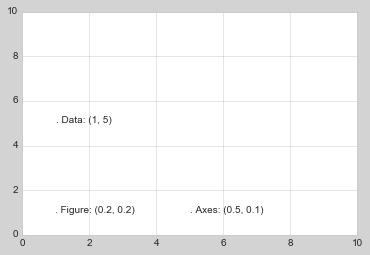Note that by default, the text is aligned above and to the left of the specified coordinates: here the "." at the beginning of each string will approximately mark the given coordinate location.

The transData coordinates give the usual data coordinates associated with the x- and y-axis labels. The transAxes coordinates give the location from the bottom-left corner of the axes (here the white box), as a fraction of the axes size. The transFigure coordinates are similar, but specify the position from the bottom-left of the figure (here the gray box), as a fraction of the figure size.

Notice now that if we change the axes limits, it is only the transData coordinates that will be affected, while the others remain stationary:

In :
ax.set_xlim(0, 2)
ax.set_ylim(-6, 6)
fig

Out: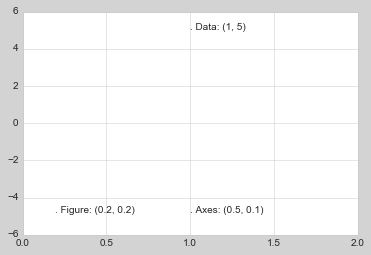This behavior can be seen more clearly by changing the axes limits interactively: if you are executing this code in a notebook, you can make that happen by changing %matplotlib inline to %matplotlib notebook and using each plot's menu to interact with the plot.

## Arrows and Annotation¶

Along with tick marks and text, another useful annotation mark is the simple arrow.

Drawing arrows in Matplotlib is often much harder than you'd bargain for. While there is a plt.arrow() function available, I wouldn't suggest using it: the arrows it creates are SVG objects that will be subject to the varying aspect ratio of your plots, and the result is rarely what the user intended. Instead, I'd suggest using the plt.annotate() function. This function creates some text and an arrow, and the arrows can be very flexibly specified.

Here we'll use annotate with several of its options:

In :
%matplotlib inline

fig, ax = plt.subplots()

x = np.linspace(0, 20, 1000)
ax.plot(x, np.cos(x))
ax.axis('equal')

ax.annotate('local maximum', xy=(6.28, 1), xytext=(10, 4),
arrowprops=dict(facecolor='black', shrink=0.05))

ax.annotate('local minimum', xy=(5 * np.pi, -1), xytext=(2, -6),
arrowprops=dict(arrowstyle="->",
connectionstyle="angle3,angleA=0,angleB=-90"));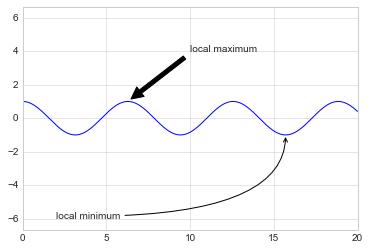The arrow style is controlled through the arrowprops dictionary, which has numerous options available. These options are fairly well-documented in Matplotlib's online documentation, so rather than repeating them here it is probably more useful to quickly show some of the possibilities. Let's demonstrate several of the possible options using the birthrate plot from before:

In :
fig, ax = plt.subplots(figsize=(12, 4))
births_by_date.plot(ax=ax)

# Add labels to the plot
ax.annotate("New Year's Day", xy=('2012-1-1', 4100),  xycoords='data',
xytext=(50, -30), textcoords='offset points',
arrowprops=dict(arrowstyle="->",

ax.annotate("Independence Day", xy=('2012-7-4', 4250),  xycoords='data',
bbox=dict(boxstyle="round", fc="none", ec="gray"),
xytext=(10, -40), textcoords='offset points', ha='center',
arrowprops=dict(arrowstyle="->"))

ax.annotate('Labor Day', xy=('2012-9-4', 4850), xycoords='data', ha='center',
xytext=(0, -20), textcoords='offset points')
ax.annotate('', xy=('2012-9-1', 4850), xytext=('2012-9-7', 4850),
xycoords='data', textcoords='data',
arrowprops={'arrowstyle': '|-|,widthA=0.2,widthB=0.2', })

ax.annotate('Halloween', xy=('2012-10-31', 4600),  xycoords='data',
xytext=(-80, -40), textcoords='offset points',
arrowprops=dict(arrowstyle="fancy",
fc="0.6", ec="none",
connectionstyle="angle3,angleA=0,angleB=-90"))

ax.annotate('Thanksgiving', xy=('2012-11-25', 4500),  xycoords='data',
xytext=(-120, -60), textcoords='offset points',
arrowprops=dict(arrowstyle="->",

ax.annotate('Christmas', xy=('2012-12-25', 3850),  xycoords='data',
xytext=(-30, 0), textcoords='offset points',
size=13, ha='right', va="center",
bbox=dict(boxstyle="round", alpha=0.1),
arrowprops=dict(arrowstyle="wedge,tail_width=0.5", alpha=0.1));

# Label the axes
ax.set(title='USA births by day of year (1969-1988)',
ylabel='average daily births')

# Format the x axis with centered month labels
ax.xaxis.set_major_locator(mpl.dates.MonthLocator())
ax.xaxis.set_minor_locator(mpl.dates.MonthLocator(bymonthday=15))
ax.xaxis.set_major_formatter(plt.NullFormatter())
ax.xaxis.set_minor_formatter(mpl.dates.DateFormatter('%h'));

ax.set_ylim(3600, 5400);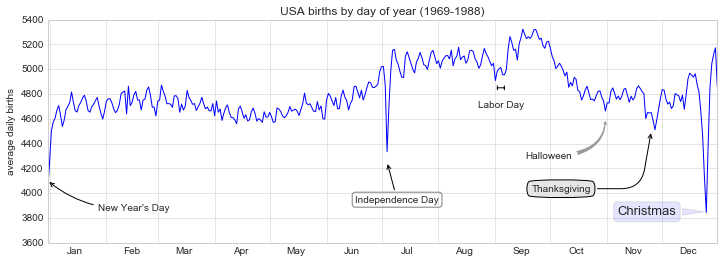You'll notice that the specifications of the arrows and text boxes are very detailed: this gives you the power to create nearly any arrow style you wish. Unfortunately, it also means that these sorts of features often must be manually tweaked, a process that can be very time consuming when producing publication-quality graphics! Finally, I'll note that the preceding mix of styles is by no means best practice for presenting data, but rather included as a demonstration of some of the available options.

More discussion and examples of available arrow and annotation styles can be found in the Matplotlib gallery, in particular the Annotation Demo.